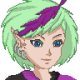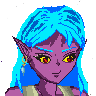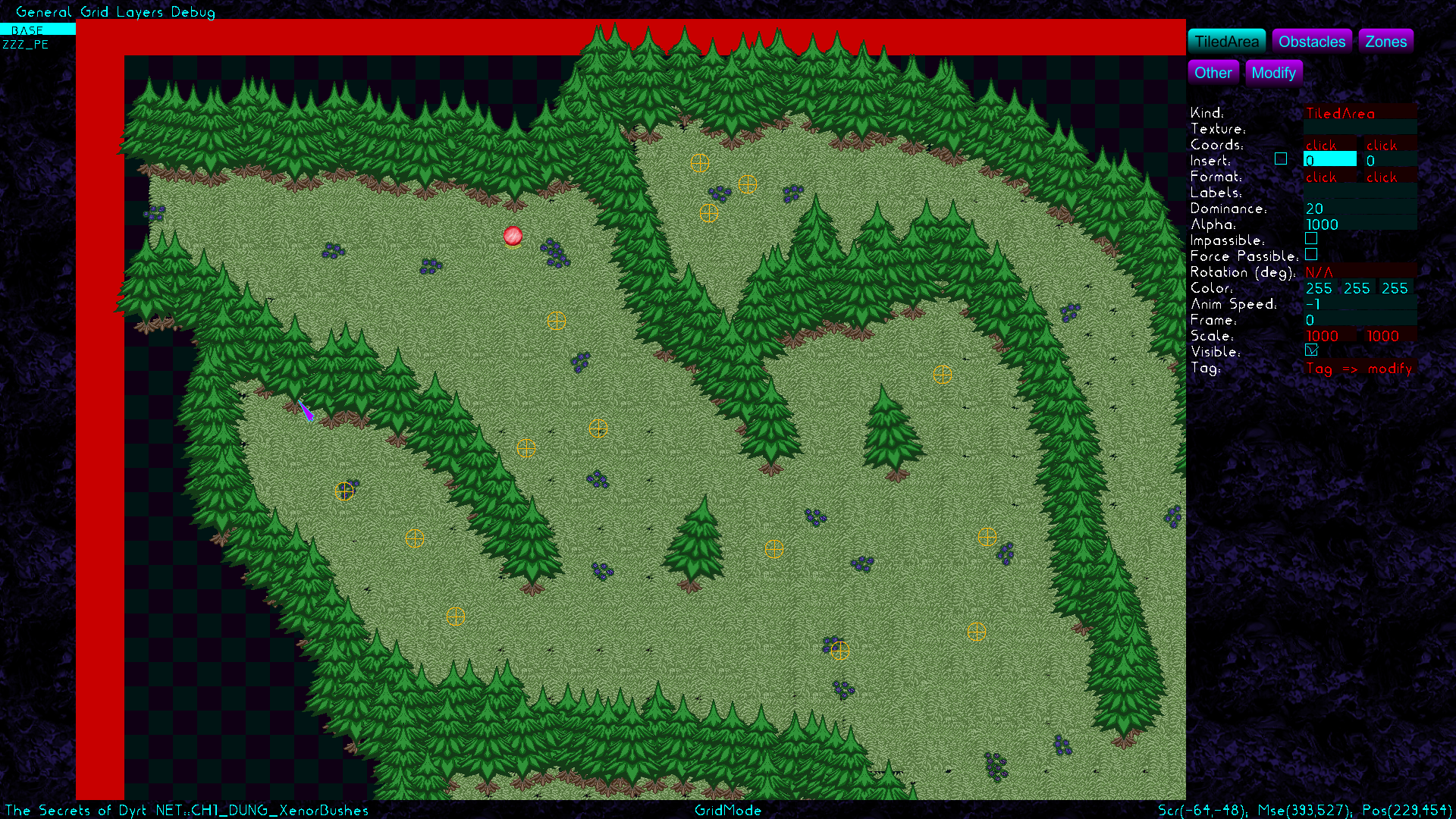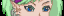-=+=- -=+=- -=+=- -=+=- -=+=- -=+=- -=+=- -=+=- -=+=- -=+=- -=+=- -=+=- -=+=- -=+=- -=+=- -=+=- -=+=- -=+=- -=+=- -=+=- -=+=- -=+=- -=+=- -=+=- -=+=- -=+=- -=+=- -=+=- -=+=- -=+=- (c) WidthPadding Industries 1987 0|505|0 -=+=- -=+=- -=+=- -=+=- -=+=- -=+=- -=+=- -=+=- -=+=- -=+=- -=+=- -=+=- -=+=- -=+=- -=+=- -=+=- -=+=- -=+=- -=+=- -=+=- -=+=- -=+=- -=+=- -=+=- -=+=- -=+=- -=+=- -=+=- -=+=- -=+=-
SoCoder -> Showcase Home -> Apps and Utilities

TrickyCreated : 24 October 2019

### Kthura - Map Editor

ScreenshotsLet's show you my Kthura Map EditorKthura is a map system for 2D Map development, but here's the rub... It's not a tile sysem, but a object oriented map system. I originally designed it for an adventure game with a girl named Kthura as main character, however the development for that game still has to seriously start... Figures. This map editor has been used in my RPG Games Star Story, and The Fairy Tale REVAMPED, and the screenshot above is taken from my current project to redo my "Secrets of Dyrt".

Kthura can do nice tricks a tile based map system cannot provide, and the screenshot above already shows a few things.... The trees are for starters not really bound to a grid, but I placed them more loosely in order to create a more natural effect, and you may even seen that I tilted two trees. I can also make them lighter, darker, transparent even. If you play the games I already created using this map system, you can also see that torches and candles are being animated in a different speed and quite often not in sync with each other. The automated doors in the Astrilopup facility of Star Story were made easy thanks to Kthura, and the secret dungeon "Subterranean river" did really benefit from Kthura in animating the river and most notable the tunnel under the water. Kthura does contain a Lua scripting engine (enhanced with NIL support) allowing me to do some special things more easily.

Kthura is not an easy tool to set up and work with, I gotta warn you for that. Took me some time to master it myself to be honest.
Kthura saves in a format that is based on JCR6, however it can export to lua, json, javascript, python and xml, however I currently only have a working engine in BlitzMax (discontinued due to issues with the compiler) and C#.

[Here's my repository and there's a release section there](https://github.com/TrickyGameTools/Kthura).